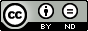#Fixed support matrix factorization is NP hard by Tung Le Quoc (ENS de Lyon) [June 28, 2021]

## Summary

GdR ISIS Théorie du deep learning - June 28, 2021

# By Tung Le Quoc (ENS de Lyon)

In this work, we contribute to the theory of neural network optimization with sparsity constraints. Consider a classical feed forward neural network where one would like to minimize the loss function while enforcing the sparsity of network (i.e, the matrix at each layer contains many zeros). Current works have to deal with two sub-problems simultaneously: finding the positions of nonzero indices (known as supports) at each layers and their corresponding coefficients. While the former can be expected to be combinatorial in nature, we show that the second one is also NP-hard even in the case of a shallow linear network (i.e. no bias, linear activation function with two layers and training data (B, A) having B = Id ). Yet, for certain families of sparsity patterns, we show that the problem becomes tractable with an efficient algorithm that can be exploited for multilayer sparse factorization.

This is a cowork by Tung Le Quoc, Elisa Riccietti, Rémi Gribonval.

## Infos

• ### Updated on:

June 29, 2021, 8:18 p.m.

00:17:35

11

English

Other
• ### Discipline(s):